# 1) Calculate the magnitude of the electric field 2.12m from a point charge of 6.4mC (such...

1) Calculate the magnitude of the electric field 2.12m from a point charge of 6.4mC (such as found on the terminal of a Van de Graaff).
??NC

2) The electric field strength between two parallel conducting plates separated by 7.0cm is 8.5x10^4 V/m. The potential difference between the plates is 6kV. The with the lowest potential is taken to he zero volts. What is the potential 1.1cm from that plate?

1) given that

charge q = 6.4*10^-3 C

distance r = 2.12 m

from the relation

electric field due to a point charge E = kq/r^2

E = 9*10^9*6.4*10^-3/2.12^2 = 12.82*10^6 N/C

2) given

plates separation d = 0.07 m

electric field E = 8.5*10^4 V/m

potential difference =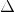V = 6*10^3 V

from the relation

change in potential = electric field*distance

v-0 = 8.5*10^4*1.1*10^-2

v = 0.935 kV

#### Earn Coin

Coins can be redeemed for fabulous gifts.

Similar Homework Help Questions
• ### Please complete both The electric field strength between two parallel conducting plates separated by 4.20 cm...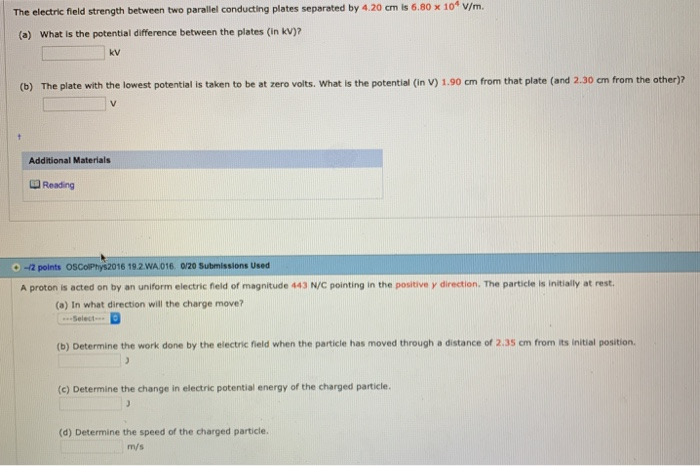Please complete both The electric field strength between two parallel conducting plates separated by 4.20 cm is 6.80 x 104 v/m. (a) What is the potential difference between the plates (in kV)2 kv (b) The plate with the lowest potential is taken to be at zero volts. What is the potential (in V) 1.90 cm from that plate (and 2.30 cm from the other)? Additional Materials Reading .-2 points oscoPhys2016 19.2.WA016 0/20 Submissions Used A proton is acted on by...

• ### QUESTION 10 Two conducting parallel plates have an electric field between them of magnitude 150 V/m,...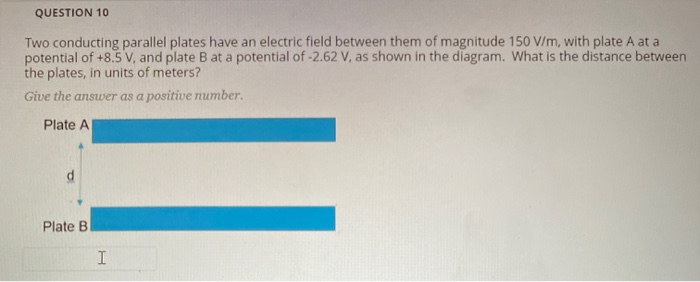QUESTION 10 Two conducting parallel plates have an electric field between them of magnitude 150 V/m, with plate A at a potential of +8.5 V, and plate B at a potential of -2.62 V, as shown in the diagram. What is the distance between the plates, in units of meters? Give the answer as a positive number. Plate A Plate B I QUESTION 9 Two conducting parallel plates have a potential difference of 21.8 V between them. If the electric...

• ### 1. My Notes -11 points OSColPhys2016 19.5.WA.040. The electric field in the region between the plates...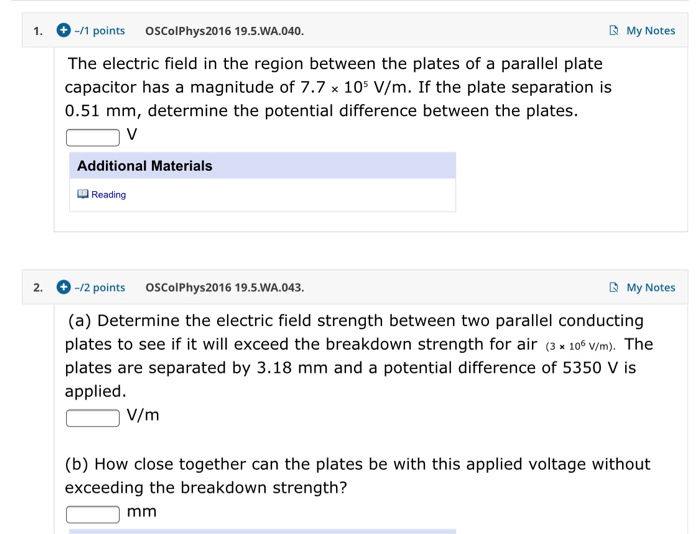1. My Notes -11 points OSColPhys2016 19.5.WA.040. The electric field in the region between the plates of a parallel plate capacitor has a magnitude of 7.7 x 105 V/m. If the plate separation is 0.51 mm, determine the potential difference between the plates. CV Additional Materials Reading 2. -/2 points OSColPhys2016 19.5.WA.043. My Notes (a) Determine the electric field strength between two parallel conducting plates to see if it will exceed the breakdown strength for air (3 x 106 V/m)....

• ### 19.17 Electric Potential in a Uniform Electric Field What is the electric field strength between two...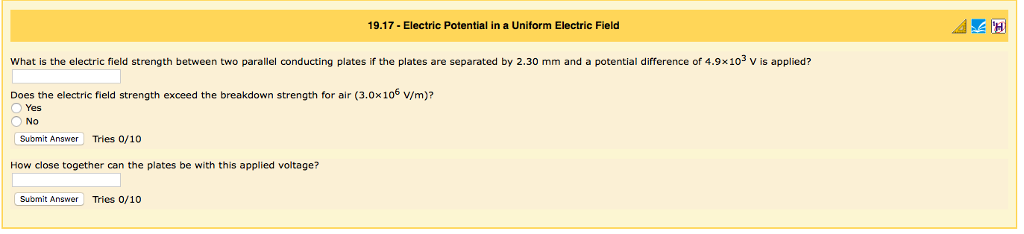19.17 Electric Potential in a Uniform Electric Field What is the electric field strength between two parallel conducting plates if the plates are separated by 2.30 mm and a potential difference of 4.9x 10 V is applied? Does the electric field strength exceed the breakdown strength for air (3.0x10° V/m)i? Yes No Submit Answer Tries o/10 How close together can the plates be with this applied voltage? Submit Answer Tries 0/10

• ### 1. A particle with a charge of +4.20 nC is in a uniform electric field E⃗  directed...

1. A particle with a charge of +4.20 nC is in a uniform electric field E⃗  directed to the negative x direction. It is released from rest, and after it has moved 6.00 cm , its kinetic energy is found to be 1.50×10−6 J . a. What work was done by the electric force? b. What was the change in electric potential over the distance that the charge moved? c. What is the magnitude of E? d. What was the change...

• ### physics

Two parallel conducting plates are separated by 10.0 cm, and one of them is taken to be at zero volts.(a) What is the electric field strength between them, if the potential 6.50 cm from the zero volt plate (and 3.50 cm from the other) is 570 V?kV/m(b) What is the voltage between the plates?V

• ### v3. At what distance from a point charge of 4.0 nC does the electric field have...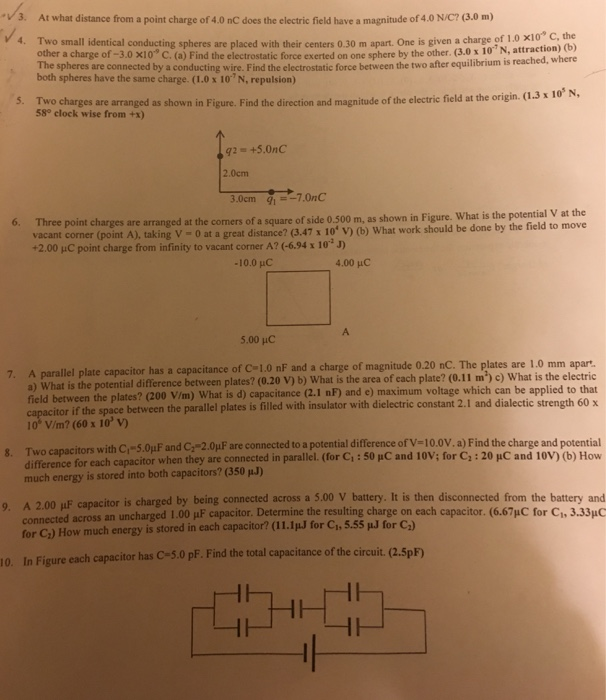v3. At what distance from a point charge of 4.0 nC does the electric field have a magnitude of 4.0 N/C? (3.0 m) 4. Two small identical conducting spheres are placed with their centers 0.30 m apart. One is given a charge of 1 0 x10* c other a charge of -3.0 x10 C. (a) Find the electrostatic force exerted on one sphere by the other. (3.0 x 10"N, attraction) the (b) e spheres are connected by a conducting wire....

• ### What is the strength of the electric field at the midpoint between two parallel conducting plates...

What is the strength of the electric field at the midpoint between two parallel conducting plates separated by a distance of 4.00 cm and maintained at a potential difference of 100 V?

• ### Will the electric field strength between two parallel conducting plates exceed the breakdown strength for air...

Will the electric field strength between two parallel conducting plates exceed the breakdown strength for air (3.0×106V/m3.0×106V/m) if the plates are separated by 2.00 mm and a potential difference of 5.0×103V5.0×103V is applied?

• ### Two large parallel conducting plates separated by 7 cm carry equal and opposite surface charge densities...

Two large parallel conducting plates separated by 7 cm carry equal and opposite surface charge densities such that the electric field between them is uniform. The difference in potential between the plates is 200 V. An electron is released from rest at the negatively charged plate.

Free Homework App